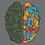# Cauchy's First Limit Theorem

If $\displaystyle \lim_{n \to \infty} a_n = 0$, then $\displaystyle \lim_{n \to \infty} \frac{a_1 + a_2 + a_3 + \dots a_n}{n} = 0$

As every convergent sequence in $\mathbb{R}$ is bounded, so there exists positive real number $K$ such that $|a_n| < K \text{ for all } n \in \mathbb{N}$

As $\displaystyle \lim_{n \to \infty} a_n = 0$, so if $\epsilon > 0$ be given, corresponding to $\epsilon$, there exists natural number $p$ such that $|a_n - 0| < \dfrac{\epsilon}2 \text{ for all } n \geq p$

$\Bigg| \dfrac{a_1 + a_2 + a_3 + \dots a_n}{n} - 0 \Bigg| \geq \frac{|a_1| + |a_2| + |a_3| + \dots |a_p|}{n} + \frac{|a_{p+1}| + |a_{p+2}| + \dots |a_n|}{n} < \dfrac{pk}n + \dfrac{\epsilon}{2n} (n-p) < \dfrac{pk}{n} + \dfrac{\epsilon}{2} \text{ for all } n \geq p.$

As $p$ and $k$ are fixed, so $\displaystyle \lim_{n \to \infty} \dfrac{pk}{n} = 0$

Corresponding to above $\epsilon > 0$, there exists a natural number $q$ such that

$\dfrac{pk}{n} < \dfrac{\epsilon}{2} \text{ for all } n \geq q$

In fact, here $q = \lfloor \dfrac{2pk}{\epsilon} \rfloor + 1$

So for $n \geq m = \text{ max(p, q)}$,

$\Bigg| \dfrac{a_1 + a_2 + a_3 + \dots a_n}{n} \Bigg| < \dfrac{\epsilon}2 + \dfrac{\epsilon}2 \implies \displaystyle \lim_{n \to \infty} \frac{a_1 + a_2 + a_3 + \dots a_n}{n} = 0$

Corollary: If we take $\displaystyle \lim_{n \to \infty} b_n = l, 0 < |l| < \infty$

Then taking $x_n = b_n - l,$ we have $x_n \to \infty$ as $n \to \infty$.

So \begin{aligned} \displaystyle \lim_{n \to \infty} \frac{x_1 + x_2 + x_3 + \dots x_n}{n} &= 0. \\ \implies \lim_{n \to \infty} \frac{b_1 + b_2 + b_3 + \dots b_n}{n} - l &= 0 \\ \implies \lim_{n \to \infty} \frac{b_1 + b_2 + b_3 + \dots b_n}{n} &= l \end{aligned}

If ${x_n}_n, x_n > 0$ for all $n$, converges to $l, l > 0,$ then show that $\sqrt[n]{x_1x_2x_3 \cdots x_n} \to l$

\begin{aligned} \text{We know that } \displaystyle \lim_{n \to \infty} x_n &= l (x_n > 0, l > 0) \\ \implies \lim_{n \to \infty} \ln x_n &= \ln l \\ \text{Using Cauchy's First Limit Theorem} \\ \lim_{n \to \infty} \frac{\ln x_1 + \ln x_2 + \dots + \ln x_n}{n} &= \ln l \\ \implies \lim_{n \to \infty} \ln \left( \sqrt[n]{x_1x_2x_3 \cdots x_n} \right) &= \ln l \\ \implies \sqrt[n]{x_1x_2x_3 \cdots x_n} \to l \text{ as } n \to \infty \end{aligned}1 month, 3 weeks ago

This discussion board is a place to discuss our Daily Challenges and the math and science related to those challenges. Explanations are more than just a solution — they should explain the steps and thinking strategies that you used to obtain the solution. Comments should further the discussion of math and science.

When posting on Brilliant:

• Use the emojis to react to an explanation, whether you're congratulating a job well done , or just really confused .
• Ask specific questions about the challenge or the steps in somebody's explanation. Well-posed questions can add a lot to the discussion, but posting "I don't understand!" doesn't help anyone.
• Try to contribute something new to the discussion, whether it is an extension, generalization or other idea related to the challenge.

MarkdownAppears as
*italics* or _italics_ italics
**bold** or __bold__ bold
- bulleted- list
• bulleted
• list
1. numbered2. list
1. numbered
2. list
Note: you must add a full line of space before and after lists for them to show up correctly
paragraph 1paragraph 2

paragraph 1

paragraph 2

[example link](https://brilliant.org)example link
> This is a quote
This is a quote
    # I indented these lines
# 4 spaces, and now they show
# up as a code block.

print "hello world"
# I indented these lines
# 4 spaces, and now they show
# up as a code block.

print "hello world"
MathAppears as
Remember to wrap math in $$ ... $$ or $ ... $ to ensure proper formatting.
2 \times 3 $2 \times 3$
2^{34} $2^{34}$
a_{i-1} $a_{i-1}$
\frac{2}{3} $\frac{2}{3}$
\sqrt{2} $\sqrt{2}$
\sum_{i=1}^3 $\sum_{i=1}^3$
\sin \theta $\sin \theta$
\boxed{123} $\boxed{123}$

Sort by:

Thanks a ton @Adhiraj Dutta . I have mentioned and linked your note in the problem I used this theorem. You can try the problem out here. I have deleted my non-Latex post on this theorem.

- 1 month, 3 weeks ago

You're welcome. Can you send the link to the question again?

- 1 month, 3 weeks ago

- 1 month, 3 weeks ago

Thank you!

- 1 month, 3 weeks ago